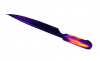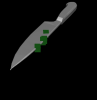# Accessing modified vertex indices in the convex mesh

Discussion in 'Priority support' started by prnk, Dec 3, 2019.

1. I am trying to load a mesh in mujoco and visualize certain vertices from the original stl mesh. However after loading the mesh, I noticed that the number of vertices has drastically increased (which I presume is the consequence of converting the mesh into a convex mesh). I would like to index a particular set of vertices from the original mesh, but since the vertex set has changed after loading, these vertices don't correspond to the ones I want anymore.

The sample script here highlights the issue. I have also attached a zip file containing the code and mesh files.

Output of the script:
Number of vertices: (204208, 3)
Number of meshes: 1
Number of vertices in all meshes: 204208
Number of vertices in each mesh: 
Starting address of each mesh: 
Number of vertices in mujoco mesh: 204208
Number of vertices in ply mesh: (59252, 3)

Number of points in stl mesh: (355500, 3)
Number of unique points in stl mesh: (59252, 3)

Fig 1. shows the original ply mesh containing 59,252 vertices. The orange regions contain the subset of vertices that I am interested in. After converting the mesh to stl and loading it in mujoco however, the number of vertices increases to 204,208. When I index the mesh using the original vertex indices, these vertices are scattered throughout the body of the mesh (Fig. 2).Is my understanding correct that the increase in number of vertices is because mujoco converts the mesh into a convex mesh?
How can I access the original vertices that have been re-indexed in the convex mesh?

#### Attached Files:

• ###### debug_mesh_verts.zip
File size:
4.8 MB
Views:
50
2. So, I noticed that mujoco duplicates a lot of vertices after processing the original mesh. Taking the unique vertex set and performing an ICP registration with the original mesh solves my vertex correspondence issue.